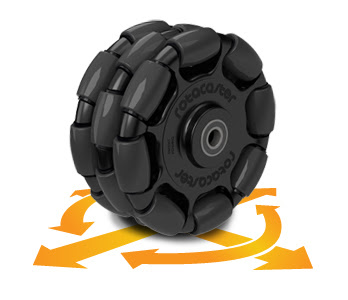Holonomic Motion Control, Omni Wheels

I've visualised holonomic motion control in Processing.  This is the type of unconstrained motion you can achieve with omni-wheels or mecanum wheels.  You can do fun things with a robot, like drive in a straight line whilst rotating the body of the robot.An omni-wheel, image from wikimedia.

In the code I have attempted to break down the kinematics into discrete steps as much as possible.  Hopefully, the code will illustrate that matrix transformations are very easy when written in a programming language.  The kinematics were interpreted from the book 'Autonomous Mobile Robots' by Siegwart and Nourbakhsh.

If you click through you can try out an interactive javascript applet, and the source code is available through OpenProcessing, or you can download a zip file here .

You can use the mouse to set a vector for the robot, and use the keys 'a' and 'z' to change the rotational velocity of the robot.

The following two extracts are the most interesting parts of the source code:
void VectorToMotorVelocities( float mag, float angle, float rotation ) {
float vx,vy;

// In each time step, we subtract the robots angular rotation
// from the desired vector, to correct for an angular momentum
// (i.e; the robot is spinning whilst it moves on a vector).
angle =  (  angle - body.a );

// Work out the X and Y components of the vector
vx =  sin( angle );
vy =  cos( angle );

// Use the angular orientation the wheels to work out
// an appropriate contribution of X and Y components
// for each wheel.
// Note, we add in a constant angular velocity (if any)
// of the robot to each wheel here
mv1 = (vx * sin( ma1 )) - (vy * cos( ma1  ) ) - rotation;
mv2 = (vx * sin( ma2 )) - (vy * cos( ma2 ) ) - rotation;
mv3 = (vx * sin( ma3  )) - (vy * cos( ma3 ) ) - rotation;

}
and:

void DoKinematics() {
int i,j;

// Wheel (C)onstraint matrix
float[][] c_matrix = new float;
c_matrix = 1 / (sqrt(3));        c_matrix = 0;                    c_matrix = - 1 / (sqrt(3));
c_matrix = 0 - (1.0 / 3.0);      c_matrix = 2.0/3.0;              c_matrix =  0 - (1.0 / 3.0);
c_matrix = -1 / (3 * wheel_sep); c_matrix = -1 / (3 * wheel_sep); c_matrix = -1 / (3 * wheel_sep);

// (V)elocity matrix, defines contributions to
// motion in the ideal situation, when the
// robot is aligned to the global reference.
float[] v_matrix = new float;
v_matrix = mv1 * wheel_radius;
v_matrix = mv2 * wheel_radius;
v_matrix = mv3 * wheel_radius;

// Store the result in r_matrix
float[] r_matrix = new float;

// Matrix Calculation
for ( i = 0; i < 3; i++ ) {
r_matrix[i] = 0;
for ( j = 0; j < 3; j++ ) {
r_matrix[i] = r_matrix[i] + (( c_matrix[i][j] * v_matrix[j] ));
}
}

// (T)ranslation matrix
// Converts between local and global reference.
float[][] t_matrix = new float;

t_matrix = cos(body.a);
t_matrix = sin(body.a);
t_matrix = 0.0;

t_matrix = sin(body.a);
t_matrix = -cos(body.a);
t_matrix = 0.0;

t_matrix = 0;
t_matrix = 0;
t_matrix = 1;

double[] result = new double;

// Matrix Calculation
for ( i = 0; i < 3; i++ ) {
result[i] = 0;
for ( j = 0; j < 3; j++ ) {
result[i] += (t_matrix[i][j] * r_matrix[j]);
}
}

//println(" Result: \t[ " + result + " ][ " + result + " ][ " + result + " ]");
//println("");
body.x += result;
body.y += result;
body.a += result;

// Constrain the co-ordinates to the screen.
body.x = constrain( body.x, -200, 200 );
body.y = constrain( body.y, -200, 200 );

}